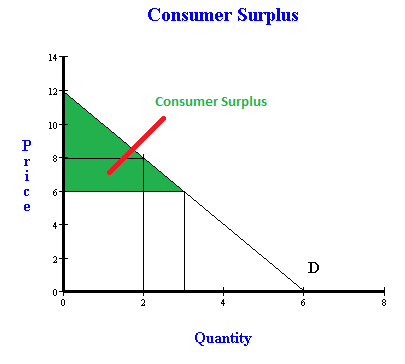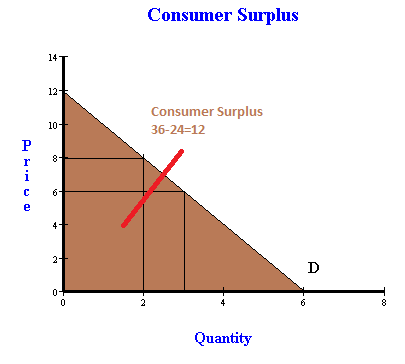Calculating consumer surplus with all you can eat - FreeEconHelp.com, Learning Economics... Solved!

## 7/17/14

This post goes over the example problem of calculating consumer surplus from different scenarios. The scenario sets up by giving you a table depicting a demand curve and then asks you to calculate consumer surplus with different prices. Finally, an all you can eat scenario is introduced where you pay a flat fee to enter the transaction but the marginal cost of each additional unit is effectively zero. Check this past post for a review of calculating consumer surplus.

A restaurant sells hot wings. A consumers demand for hotwings can be shown as:
Number of hot wing servings and the willingness to pay for hotwings (each serving)

1 \$10
2 \$8
3 \$6
4 \$4
5 \$2
6 \$0

a. If the price of an additional serving of hotwings is \$6, how many servings will be purchased? How much consumer surplus does will you receive?Consumer Surplus with a price of \$6
The information from the table tells us that we have a downward sloping demand curve that intersects the price axis at a price of \$12, and the quantity axis at a quantity of 6. If we assume a price of \$6 as in (a) then we will get a quantity of 3. Consumer surplus can be calculated by looking that the triangle that is below the demand curve but above the price. For (a) the triangle will have a height of 6, and a base of 3. Multiplying base times height and dividing by two gives us \$9. You will receive consumer surplus equal to \$9 with this demand curve and a price of \$6.

b. Imagine the price of a serving of hotwings is \$8. By how much does your consumer surplus decrease compared to the previous week?Consumer Surplus with a price of \$8
If the price goes up to \$8 per serving, then we use the same process but have a smaller triangle. In this scenario, the height of the consumer surplus triangle will be \$4, and the base 2. This gives us an area of \$4, so your consumer surplus would be equal to \$4.

c. One week later, you return to the restaurant again. You discover that the restaurant is offering an “all-you-can-eat” special for \$24. How many hotwings will you eat now and how much consumer surplus do you receive now?Consumer Surplus with all you can eat.
In this scenario, you pay \$24 for all you can eat hotwings. Since the marginal cost of each additional hotwing is 0, the price of hotwings is effectively zero. However, you have to pay \$24 to get the marginal price to be 0. If the marginal price is 0, you will consume six servings. The height of the triangle is \$12, and the base is 6. The area will be the entire triangle underneath the demand curve. This comes out to 12*6/2 or 36. Your consumer surplus in this situation would be \$36.However, since you had to pay \$24 to get this deal, your net consumer surplus would be that area minus \$24, and \$36-\$24=\$12

d. Suppose you own the restaurant and your demand curve represents a “typical” customer. What is the highest price you can charge for the “all-you-can-eat” special and still attract customers?

Finally, we can answer (d) using information from (c). If your consumer surplus is \$36, the maximum you would be able to charge and still have them take the deal is \$36. However, at this price people would be indifferent between doing the deal or not, so it would make more sense to charge \$35.99 in order to ensure they take the deal (and have positive consumer surplus).